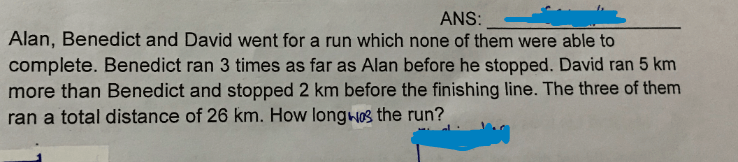# Question1. Appreciate any help to the above question

Alan : 1 unit
Benedict : 3 units
David : 3 units + 5
Total : 7 units + 5 = 26
1 unit = (26 – 5)/7 = 3
3 units + 5 + 2 = 3 x 3 + 5 + 2 = 16

Ans : 16 km.